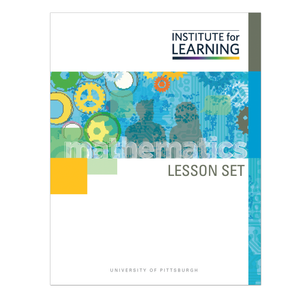# Multiplication with Fractions: Finding Portions of Numbers (Grade 5)

Regular price \$6.25 \$0.00 Unit price per

This lesson set provides a study of multiplication when one or both factors are fractions. Students will apply the meaning of the factors (number of groups and number in each group) to numbers less than 1. They will use visual representations and reasoning about numbers to understand the concept as well as the traditional algorithm. Students will:

• solve contextual situations using a variety of strategies;
• determine that all visual models of fractions can be used to represent fractional multiplication;
• recognize that repeated addition can be used for some, but not all, fractional multiplication problems; and
• reason about why the product in fractional multiplication is less than one or both factors.

Standards: 5.NF.B.4, 5.NF.B.4.A, 5.NF.B.4.B, 5.NF.B.5, 5.NF.B.5.B, 5.NF.B.6